Up: Time Dependent Fluctuations Previous: Relaxation & Time Correlation

Subsections

# Thermodynamic Forces & Kinetic Coefficients. Onsager Law

## Kinetic Coefficients

Thermodynamic force:
We introduced before conjugated variables: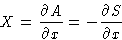Let us set zero at the equilibrium value: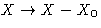. This is called thermodynamic force.
Examples:
If x is volume, the force is X=-(P-P0), if x is the number of particles,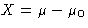, etc.

In equilibrium (x=0) we have: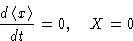When x>0,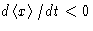and X>0: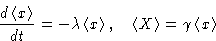From these equations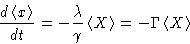(9)
The new variable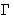is called kinetic (or Onsager) coefficient. We have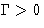(why?).

Two ways to create a fluctuation:

1.
Apply an external force X
2.
Wait long enough, and it will happenEquation (9) shows that these two ways are equivalent. No matter how we created a fluctuation, it will relax in the same way.

## Multi-Variate Case. Onsager Law

Suppose we have many variables xi. We have many thermodynamic forces Xi. Equations for relaxation:Onsager Law:
The coefficients are symmetric: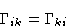This equation can be proved from the symmetry with respect to time inversion: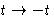.Up: Time Dependent Fluctuations Previous: Relaxation & Time Correlation

© 1997 Boris Veytsman and Michael Kotelyanskii
Tue Oct 28 22:13:33 EST 1997